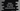# Python program to sort values of one list using second list## Python program to sort one list by using values of a different list :

In this example program, we will learn how to sort elements of one list by using elements of a different list.

It looks confusing, don’t you think? How can we sort one list using a different list?

Actually, our program will have two lists of equal lengths. All values of the first list will be mapped to the second list, or the program will assume that the elements of the first list will always be in the same position as the second list. For example, if we move the first element of the first list to the third position, the first element of the second list will move to the third position.

Python list is a collection used to store different or same datatypes or objects. Python list is an ordered collection and it is mutable. Each item in a list can be accessed by using its index. The index of the list starts from 0, i.e. the index of the first item is 0, the second item is 1 etc.

Square bracket [ ] is used for python list. All items are placed inside a square bracket separating each one by a comma. We can access any item using its index like mylist[i], where _mylist_ is our list and i is the index we want to access. This will return the item on index i of the list mylist_.

### Algorithm :

For sorting one list using a second list, we need to put them both in a single list. We will use zip() method for it. This is an inbuilt python method and it returns a list of tuple. If we pass two lists as its first and the second argument, it will return a list of tuple with item pair of the lists.

The first element of the list will be a tuple with its first element as the first element of the first list and second element as the first element of the second list. Similarly, all other items of both lists will be put in a tuple in the final list.

Note that if the first list is of length 3 and the second list of length 4, the final tuple list will have only 3 elements. The last element of the second list will be ignored. In this example, we are taking two lists of equal length.

After this step, we can sort the tuple list easily by using the sorted( ) method. It will sort the list by considering the first elements of all tuples. Or it will sort the first list of items. All other items of the second list will also be sorted based on the first list items.

Let’s take a look at the program :

### Python Program:

``````first_list = ["O","X","A","C","D","K"]
second_list = ['1','2','3','4','5','6']

zipped_pairs = zip(first_list,second_list)

sorted_pairs = sorted(zipped_pairs)

result = [item for item in sorted_pairs]

print(result)``````

You can copy the above code from here.

### Explanation:

Here, we have two list firstlist_ and secondlist. We are sorting the values of secondlist using first_list. The output of the program is :

``['3', '4', '5', '6', '1', '2']``

Since the value 3 is on the third position of secondlist_ and ‘A’ is on the third position of firstlist, it is placed in the first position. Similarly, the other elements are also placed by comparing with the elements of _firstlist_.